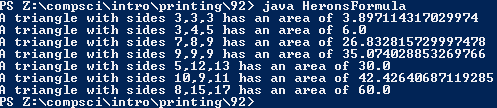# Programming Assignment #92

## Code

```
// Yes they output the same thing.
// HeronsFormula is only 35 lines while HeronsFormulaNoMethod is 50 lines.
// It was easier to change it in this file because there was less to change.
// It was also very easy to add.

public class HeronsFormula
{
public static void main( String[] args )
{
double a;

a = triangleArea(3, 3, 3);
System.out.println("A triangle with sides 3,3,3 has an area of " + a );

a = triangleArea(3, 4, 5);
System.out.println("A triangle with sides 3,4,5 has an area of " + a );

a = triangleArea(7, 8, 9);
System.out.println("A triangle with sides 7,8,9 has an area of " + a );

System.out.println("A triangle with sides 9,9,9 has an area of " + triangleArea(9, 9, 9) );

System.out.println("A triangle with sides 5,12,13 has an area of " + triangleArea(5, 12, 13) );
System.out.println("A triangle with sides 10,9,11 has an area of " + triangleArea(10, 9, 11) );
System.out.println("A triangle with sides 8,15,17 has an area of " + triangleArea(8, 15, 17) );
}

public static double triangleArea( int a, int b, int c )
{
// the code in this method computes the area of a triangle whose sides have lengths a, b, and c
double s, A;

s = (a+b+c) / 2.0;
A = Math.sqrt( s*(s-a)*(s-b)*(s-c) );

return A;
// ^ after computing the area, "return" it
}
}

```

## Outputs